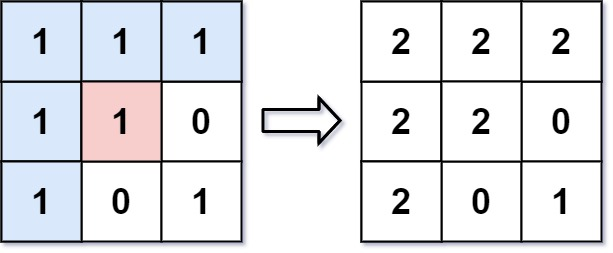## Algorithm

Problem Name: 733. Flood Fill

An image is represented by an `m x n` integer grid `image` where `image[i][j]` represents the pixel value of the image.

You are also given three integers `sr`, `sc`, and `color`. You should perform a flood fill on the image starting from the pixel `image[sr][sc]`.

To perform a flood fill, consider the starting pixel, plus any pixels connected 4-directionally to the starting pixel of the same color as the starting pixel, plus any pixels connected 4-directionally to those pixels (also with the same color), and so on. Replace the color of all of the aforementioned pixels with `color`.

Return the modified image after performing the flood fill.

Example 1:```Input: image = [[1,1,1],[1,1,0],[1,0,1]], sr = 1, sc = 1, color = 2
Output: [[2,2,2],[2,2,0],[2,0,1]]
Explanation: From the center of the image with position (sr, sc) = (1, 1) (i.e., the red pixel), all pixels connected by a path of the same color as the starting pixel (i.e., the blue pixels) are colored with the new color.
Note the bottom corner is not colored 2, because it is not 4-directionally connected to the starting pixel.
```

Example 2:

```Input: image = [[0,0,0],[0,0,0]], sr = 0, sc = 0, color = 0
Output: [[0,0,0],[0,0,0]]
Explanation: The starting pixel is already colored 0, so no changes are made to the image.
```

Constraints:

• `m == image.length`
• `n == image[i].length`
• `1 <= m, n <= 50`
• `0 <= image[i][j], color < 216`
• `0 <= sr < m`
• `0 <= sc < n`

## Code Examples

### #1 Code Example with C++ Programming

```Code - C++ Programming```

``````
class Solution {
public:
vector> floodFill(vector>& image, int sr, int sc, int newColor) {
int m = image.size(), n = image.size();
DFS(image, sr, sc, m, n, image[sr][sc], newColor);
return image;
}

void DFS(vector>& image, int r, int c, int m, int n, int target, int newColor){
if(r < 0 || c < 0 || r == m || c == n || image[r][c] == newColor || image[r][c] != target) return;
image[r][c] = newColor;
DFS(image, r + 1, c, m, n, target, newColor);
DFS(image, r - 1, c, m, n, target, newColor);
DFS(image, r, c + 1, m, n, target, newColor);
DFS(image, r, c - 1, m, n, target, newColor);
}
};
``````
Copy The Code &

Input

cmd
image = [[1,1,1],[1,1,0],[1,0,1]], sr = 1, sc = 1, color = 2

Output

cmd
[[2,2,2],[2,2,0],[2,0,1]]

### #2 Code Example with Java Programming

```Code - Java Programming```

``````
class Solution {
private static final int[][] DIRS = {{0, 1}, {1, 0}, {0, -1}, {-1, 0}};

public int[][] floodFill(int[][] image, int sr, int sc, int newColor) {
int numOfRows = image.length;
int numOfCols = image.length;
int originalColor = image[sr][sc];
if (newColor == originalColor) {
return image;
}
while (!queue.isEmpty()) {
int size = queue.size();
while (size-- > 0) {
int[] coordinate = queue.remove();
int xPoint = coordinate;
int yPoint = coordinate;
image[xPoint][yPoint] = newColor;
for (int[] dir : DIRS) {
int newXPoint = xPoint + dir;
int newYPoint = yPoint + dir;
if (newXPoint >= 0 && newYPoint >= 0 && newXPoint < numOfRows && newYPoint < numOfCols && image[newXPoint][newYPoint] == originalColor) {
}
}
}
}
return image;
}
}
``````
Copy The Code &

Input

cmd
image = [[1,1,1],[1,1,0],[1,0,1]], sr = 1, sc = 1, color = 2

Output

cmd
[[2,2,2],[2,2,0],[2,0,1]]

### #3 Code Example with Javascript Programming

```Code - Javascript Programming```

``````
const floodFill = function(image, sr, sc, newColor, firstColor = image[sr][sc]) {
if (
sr < 0 ||
sc < 0 ||
sr >= image.length ||
sc >= image[sr].length ||
image[sr][sc] !== firstColor ||
image[sr][sc] === newColor
) {
return image
}

image[sr][sc] = newColor

floodFill(image, sr + 1, sc, newColor, firstColor)
floodFill(image, sr - 1, sc, newColor, firstColor)
floodFill(image, sr, sc + 1, newColor, firstColor)
floodFill(image, sr, sc - 1, newColor, firstColor)

return image
}
``````
Copy The Code &

Input

cmd
image = [[0,0,0],[0,0,0]], sr = 0, sc = 0, color = 0

Output

cmd
[[0,0,0],[0,0,0]]

### #4 Code Example with Python Programming

```Code - Python Programming```

``````
class Solution:
def floodFill(self, image, sr, sc, newColor):
old, m, n = image[sr][sc], len(image), len(image)
if old != newColor:
q = collections.deque([(sr, sc)])
while q:
i, j = q.popleft()
image[i][j] = newColor
for x, y in ((i - 1, j), (i + 1, j), (i, j - 1), (i, j + 1)):
if 0 <= x < m and 0 <= y < n and image[x][y] == old:
q.append((x, y))
return image
``````
Copy The Code &

Input

cmd
image = [[0,0,0],[0,0,0]], sr = 0, sc = 0, color = 0

Output

cmd
[[0,0,0],[0,0,0]]

### #5 Code Example with C# Programming

```Code - C# Programming```

``````
using System.Collections.Generic;

namespace LeetCode
{
public class _0733_FloodFill
{
public int[][] FloodFill(int[][] image, int sr, int sc, int newColor)
{
var oldColor = image[sr][sc];
if (oldColor == newColor) return image;

var rows = image.Length;
var cols = image.Length;
var queue = new Queue<(int row, int col)>();
queue.Enqueue((sr, sc));
while (queue.Count > 0)
{
(int row, int col) = queue.Dequeue();
image[row][col] = newColor;

if (row > 0 && image[row - 1][col] == oldColor) queue.Enqueue((row - 1, col));
if (row < rows - 1 && image[row + 1][col] == oldColor) queue.Enqueue((row + 1, col));
if (col > 0 && image[row][col - 1] == oldColor) queue.Enqueue((row, col - 1));
if (col < cols - 1 && image[row][col + 1] == oldColor) queue.Enqueue((row, col + 1));
}

return image;
}
}
}
``````
Copy The Code &

Input

cmd
image = [[1,1,1],[1,1,0],[1,0,1]], sr = 1, sc = 1, color = 2

Output

cmd
[[2,2,2],[2,2,0],[2,0,1]]